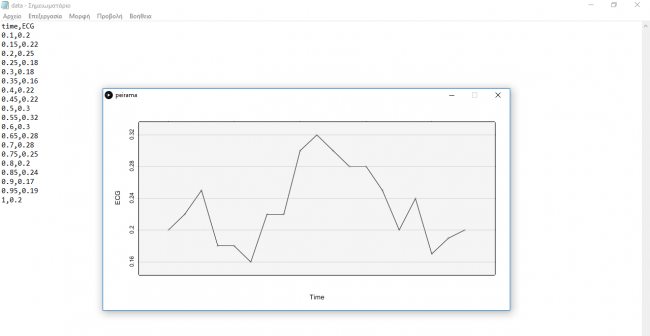#### Howdy, Stranger!

We are about to switch to a new forum software. Until then we have removed the registration on this forum.

# How can i numerate x axis in a graph?

edited January 2018

This code bellow is about making a graph from, loading a csv file.My only problem is that i can not numerate the x axis, as you see in the picture of my graph bellow.But my y axis was numerated automatically.

``````import grafica.*;

GPlot plot;

Table table;

void setup() {
size(800, 410);
table.setColumnType("time", Table.FLOAT);
table.setColumnType("ECG", Table.FLOAT);

GPointsArray points = new GPointsArray();
float[] pointSizes = new float[table.getRowCount()];
for (int row = 0; row < table.getRowCount(); row++) {
Float time = table.getFloat(row,"time");
Float ECG = table.getFloat(row,"ECG");

pointSizes[row] =5;
}
plot = new GPlot(this);
plot.setPos(0, 0);
plot.setDim(700, 300);
plot.setMar(60, 70, 40, 70);
plot.getXAxis().setNTicks(0);
plot.setPoints(points);
plot.setPointColor(color(100, 100, 255, 50));
plot.setPointSizes(pointSizes);
plot.getYAxis().setAxisLabelText("ECG");
plot.getXAxis().setAxisLabelText("Time");
plot.setAxesOffset(1);
plot.setTicksLength(1);

}
void draw() {
background(255);

// Draw the plot
plot.beginDraw();
//plot.defaultDraw();
plot.drawBackground();
plot.drawBox();
plot.drawXAxis();
plot.drawYAxis();
plot.drawTopAxis();
plot.drawRightAxis();
plot.drawTitle();
plot.drawPoints();
plot.drawLines();
plot.drawGridLines(GPlot.BOTH);
plot.drawLines();
plot.drawLabels();
//plot.drawGridLines(GPlot.VERTICAL);
//plot.drawFilledContours(GPlot.HORIZONTAL, 0);
plot.endDraw();
}
``````Tagged:

• Not sure how this library works but in setup you have this for the x-axis but not for the y

plot.getXAxis().setNTicks(0);

Maybe comment out this line

As we discussed for the graph itself: it could also be that the data is not suitable.

Maybe float is wrong

In the version above I don’t see the innovations I brought in at all. Maybe my sketch works?

• Thank you so much for your help.!!! I am grateful to you!!!

Yes, you are right!!! Th wrong line was plot.getXAxis().setNTicks(0);

Now i have numerated x- axis but i cant understand the numbers..I thin that the numbers in x-axis are wrong..